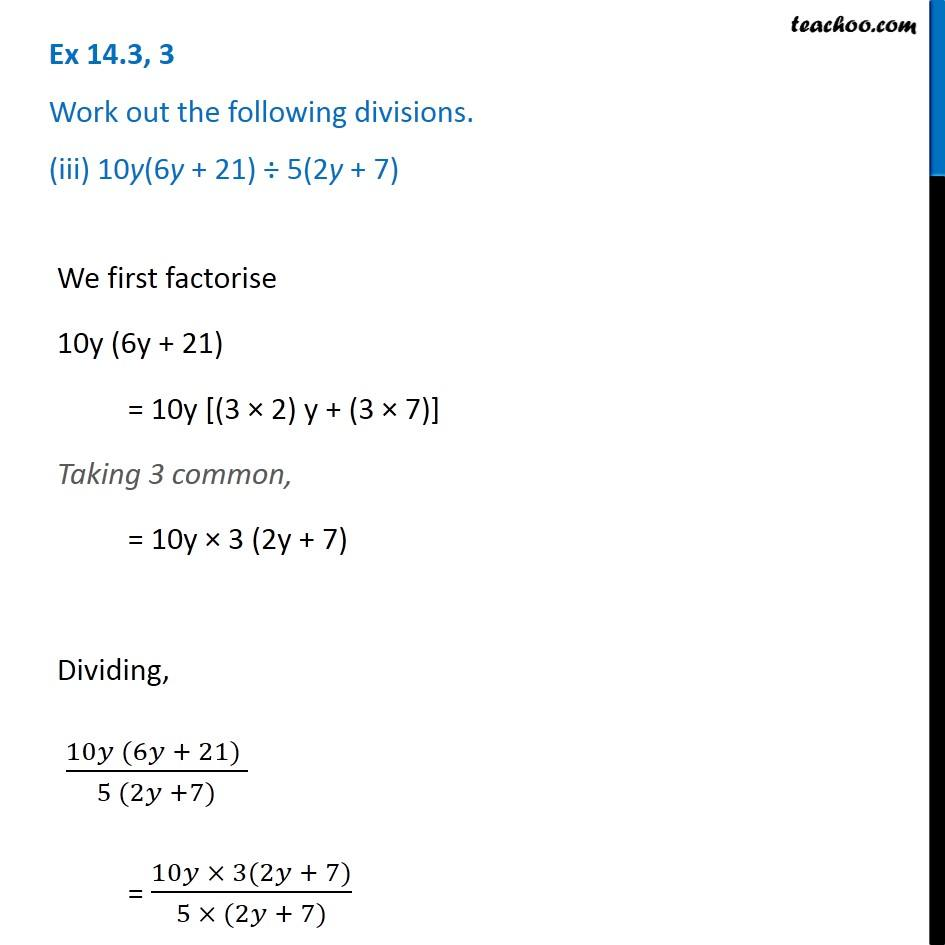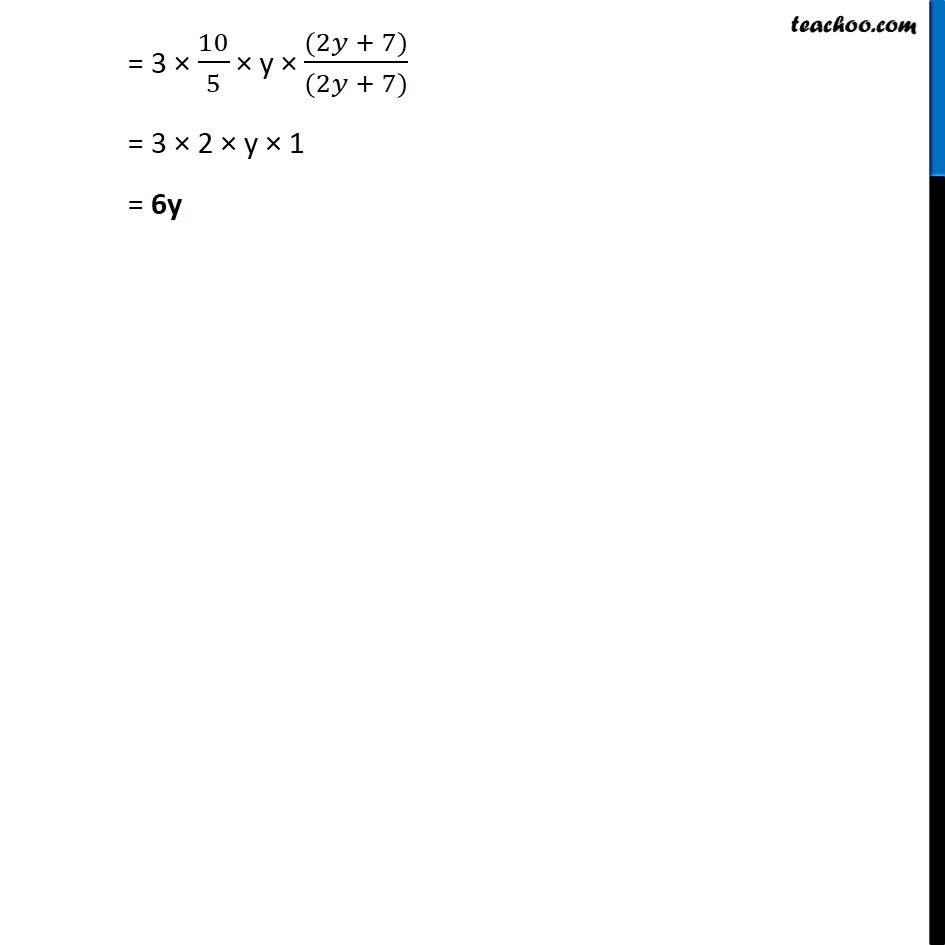Ex 14.3

Chapter 14 Class 8 Factorisation
Serial order wiseGet live Maths 1-on-1 Classs - Class 6 to 12

### Transcript

Ex 14.3, 3 Work out the following divisions. (iii) 10y(6y + 21) ÷ 5(2y + 7) We first factorise 10y (6y + 21) = 10y [(3 × 2) y + (3 × 7)] Taking 3 common, = 10y × 3 (2y + 7) Dividing, (10𝑦 (6𝑦 + 21) )/(5 (2𝑦 +7)) = (10𝑦 × 3(2𝑦 + 7))/(5 × (2𝑦 + 7)) = 3 × 10/5 × y × ((2𝑦 + 7))/((2𝑦 + 7)) = 3 × 2 × y × 1 = 6y